# ISEE Middle Level Math : Algebraic Concepts

## Example Questions

### Example Question #51 : Algebraic Concepts

Simplify: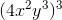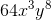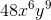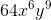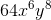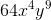Explanation:

We can first apply the power of a product rule and then apply the power of a power rule. So we can write: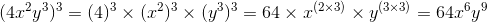### Example Question #52 : Algebraic Concepts

Simplify: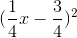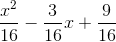None of these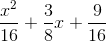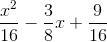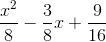Explanation:

We can solve it using the pattern for the square of a binominal or by using FOIL: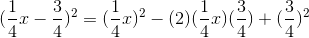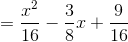### Example Question #53 : Algebraic Concepts

Simplify: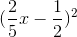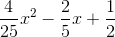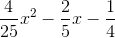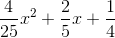None of these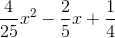Explanation:

We can solve it using the pattern for the square of a binominal or by using FOIL: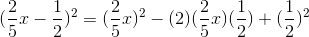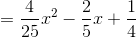### Example Question #54 : Algebraic Concepts

2 rabbits give birth to a litter of 8 rabbits. Each pair in that litter creates 4 rabbits. How many rabbits are there total, including the original 2 rabbits and their 8 children?Explanation:

If there are 8 rabbits, then that means there are 4 pairs of rabbits.

If each pair gives birth to 4 rabbits, that means there will be 16 rabbits produced by the litter (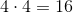).

Thus, the total number of rabbits is equal to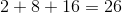Thus, 26 is the correct answer.

### Example Question #55 : Algebraic Concepts

At Bess's candy warehouse, she charges M for the first 5 pounds of candy that customers buy and P for each additional pound of candy. What is the cost of buying 128 ounces of candy from Bess's warehouse? (*Note: there are 16 ounces in a pound.)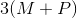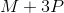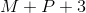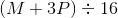Explanation:

The first step is the convert ounces into pounds. Given that there are 16 ounces in a pound, there are 8 pounds in 128 ounces because 128 divided by 16 eequals 8.

Since the first 5 pounds cost M, and each additional pound costs P, there are 3 additional pounds that will have to be paid for at the P price per pound. Thus, the appropriate formula to express the value of the cost of 128 ounces of candy (8 pounds) is:### Example Question #56 : Algebraic Concepts

Which of the following phrases can be written as the algebraic expression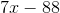?

Seven multiplied by the difference of eighty-eight and a number

Eighty-eight subtracted from the product of seven and a number

The product of seven and a number subtracted from eighty-eight

Seven multiplied by the difference of a number and eighty-eight

The correct answer is not given among the other responses

Eighty-eight subtracted from the product of seven and a number

Explanation:is eighty-eight subtracted from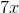.is the product of seven and a number.

Subsequently,is "eighty-eight subtracted from the product of seven and a number."

### Example Question #57 : Algebraic Concepts

Suppose you know the values of all variables in the expression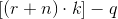and you want to evaluate the expression.

In which order will you carry out the operations?

Explanation:

By the order of operations, any expressions within grouping symbols, such as parentheses and brackets, are carried out from the inside outward. Therefore, the operation in the innermost set of grouping symbols - the addition within parentheses - will be carried out first, followed by the remaining operation within the brackets - the multiplication. The remaining operation - the subtraction - is last.

### Example Question #58 : Algebraic Concepts

Solve forin this equation: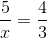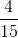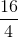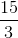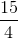Explanation:

In order to solve for, cross multiplication must be used.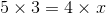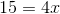Next, each side must be divided by 4. This results in: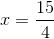### Example Question #59 : Algebraic Concepts

Multiply in modulo 7: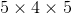Explanation:

In modulo 7 arithmetic, a number is congruent to the remainder of the divison of that number by 7. Since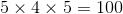and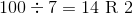then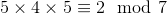.

The correct response is 2.

### Example Question #60 : Algebraic Concepts

Simplify: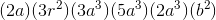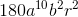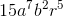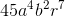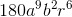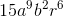Explanation:

Begin by moving all of the related variables (and constants) next to each other. You can group these in parentheses to make it clear. This is allowed because of the associative rule for multiplication.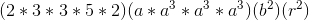When multiplying variables of the same type, you add their exponents together. This gets you: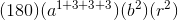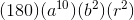This is the same as:### All ISEE Middle Level Math Resources